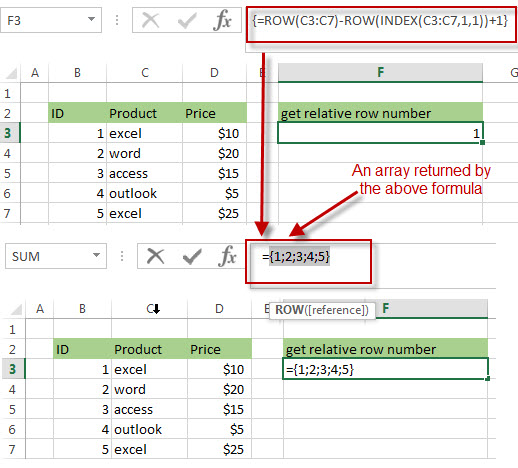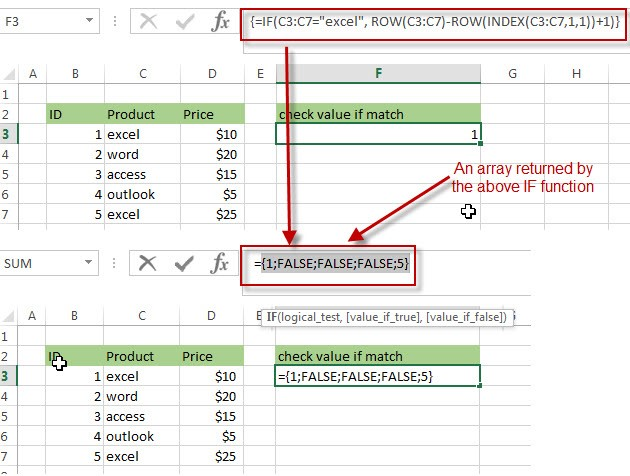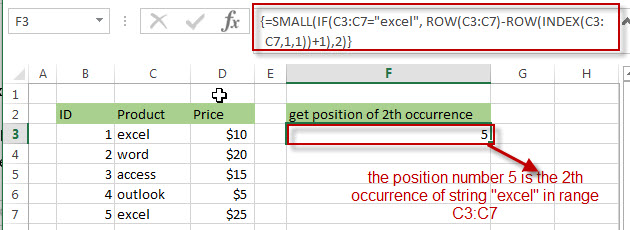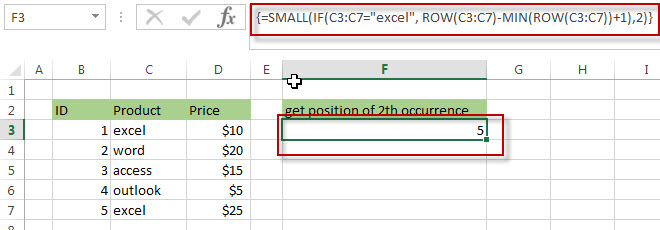# How to get the position of nth occurrence of a value in column

In previous post, we talked that how to get the position of the last occurrence of a value. And this post explains that how to get the position of the 2th, 3th or nth occurrence of a specific value in a column in excel. The below will guide you how to find the position of the nth occurrence of a value from a list in a column.

## Get the position (index) of nth Occurrence of a value

If you want to locate the second, third or nth occurrence of a specified value, you can use a combination of the INDEX function, the IF function, ROW function and SMALL function to create a new excel array formula.

For example, you want to get the position of the 2th occurrence of a string value as “excel” in a range C3:C7, you can try to use the following array formula:

`=SMALL(IF(C3:C7="excel", ROW(C3:C7)-ROW(INDEX(C3:C7,1,1))+1),2)`

Note: when you enter the above array formula, you must hold the Control and Shift keys down, then press Enter. Then the formula bar should add curly braces surrounding the array formula.

Let’s see how the above formula works:

=ROW(C3:C7)-ROW(INDEX(C3:C7,1,1))+1

I have talked the above formula in the previous post, it returns the relative position of all rows in the range C3:C7. The result returned by the above formula is an array.=IF(C3:C7=”excel”, ROW(C3:C7)-ROW(INDEX(C3:C7,1,1))+1)

The IF function will check if each value in range C3:C7 is equal to “excel”, if TRUE, returns its relative position, otherwise, returns FALSE. As this formula is an array formula, so it must be returned an array result.=SMALL(IF(C3:C7=”excel”, ROW(C3:C7)-ROW(INDEX(C3:C7,1,1))+1),2)

The SMALL function will check the array result returned by the IF function and returns the second smallest value in the array. So it should be the position of the second occurrence of text string “excel” in range C3:C7. It is 5.You can also use the below array formula to achieve the same results:

`=SMALL(IF(C3:C7="excel", ROW(C3:C7)-MIN(ROW(C3:C7))+1),2)`So if you want to get the nth occurrence of a value in a range, you just need to update the second argument of the small function to your actual requirement in the above array formula.

### Related Formulas

• Get the Position of the nth Occurrence of a Character in a Cell
If you want to get the position of the nth occurrence of a character using a excel formula, you can use the FIND function in combination with the SUBSTITUTE function.
•  Get the position of Last Occurrence of a character or string in a cell
If you want to get the position of the last occurrence of a character in a cell, then you can use a combination of the LOOKUP function, the MID function, the ROW function, the INDIRECT function and the LEN function to create an excel formula.…
• Find the Relative Position in a Range or Table
If you want to know the relative row position for all rows in an Excel Range (B3:D6), you can use a excel Array formula as follows:=ROW(B3:D6)- ROW(B3) + 1. You can also use another excel array formula to get the same result as follows:=ROW(B3:D6)-ROW(INDEX(B3:D6,1,1))+1…
• Get the First Row Number in a Range
If the ROW function use a Range as its argument, it only returns the first row number.You can also use the ROW function within the MIN function to get the first row number in a range. You can also use the INDEX function to get the reference of the first row in a range, then combined to the ROW function to get the first row number of a range.…
•  Get the Last Row Number in a Range
If you want to get the last row number in a range, you need to know the first row number and the total rows number of a range, then perform the addition operation, then subtract 1, the last result is the last row number for that range.…
• Get the position of Last Occurrence of a value in a column
If you want to find the position number of the last occurrence of a specific value in a column (a single range), you can use an array formula with a combination of the MAX function, IF function, ROW function and INDEX Function.

### Related Functions

• Excel SMALL function
The Excel SMALL function returns the smallest numeric value from the numbers that you provided. Or returns the smallest value in the array.The syntax of the SMALL function is as below:=SMALL(array,nth) …
• Excel INDEX function
The Excel INDEX function returns a value from a table based on the index (row number and column number)The INDEX function is a build-in function in Microsoft Excel and it is categorized as a Lookup and Reference Function.The syntax of the INDEX function is as below:= INDEX (array, row_num,[column_num])…
• Excel ROW function
The Excel ROW function returns the row number of a cell reference.The ROW function is a build-in function in Microsoft Excel and it is categorized as a Lookup and Reference Function.The syntax of the ROW function is as below:= ROW ([reference])….
• Excel MIN function
The Excel MIN function returns the smallest numeric value from the numbers that you provided. Or returns the smallest value in the array.The MIN function is a build-in function in Microsoft Excel and it is categorized as a Statistical Function.The syntax of the MIN function is as below:= MIN(num1,[num2,…numn])….
• Excel IF function
The Excel IF function perform a logical test to return one value if the condition is TRUE and return another value if the condition is FALSE. The IF function is a build-in function in Microsoft Excel and it is categorized as a Logical Function.The syntax of the IF function is as below:= IF (condition, [true_value], [false_value])….

Related Posts

Extract matching values From Two Lists

Suppose that you are working with two lists containing few values, and you want to extract the matching values from those two lists into another separate list. You might prefer to manually extract the matching values from the two lists, ...

Extract all Partial Matches

Just assume that you have a range of data that contains a text string and you want to extract the few partial matches into another separate range of cells; then you might think that it's not a big deal; because ...

Extract Multiple Match Values into Separate Columns

If you have a few values/items in the excel sheet and you are thinking that with the aid of the “VlOOKUP” function you can look for a specific value, extract it and then put the matching item into the separate ...

How to Sum the Smallest N Values in Excel

Sometimes we may want to sum the first smallest N numbers in a range in Excel. In this article, we will show you the method of “SUM the Smallest N Numbers” by a simple formula which consist of SUMPRODUCT and ...

How to Create Dynamical Drop-Down List and Sort by Alphabetical Order in Excel

In our daily work we may need to create a dynamical dropdown list and sort all values by alphabetical order. To create a dropdown list like this, we need to apply some built-in features like ‘Define Name’ and ‘Data Validation’ ...

How to Find the Smallest Value and Smallest Positive Value in Excel

Sometimes we want to find out the smallest positive value among a set of values. We should ignore the negative value and zero value when getting the smallest positive value. So we design different cases in this article to demonstrate ...

How to Get Row Number From a Vlookup in Excel

This post will guide you how to get row number from a Vlookup in Excel. How do I return a row number from a Vlookup with a formula in Excel. Assuming that you have a list of data in range ...

How to Get the First, 2nd or Nth Match Using Vlookup/Index/Match

This post will guide you how get the nth matching values with VLOOKUP function in Excel. How do I find the nth match value with Index/Match formula in Excel. How to find the first, second, third or nth matching value ...

Ignoring Blank or Zero Cells with Conditional formatting

This post will guide you how to make conditional formatting ignore blank cells or zero cell in Excel. How do I force blank cells or zero cells to be ignored in conditional formatting in Excel. How to ignore blank cells ...

Find the Earliest and Latest Date in a Range of Dates in Excel

This post will guide you how to find the earliest date in a range of dates in Excel. How do I get the earliest and latest date in a range with a formula in Excel. How to find the highest ...

Sidebar